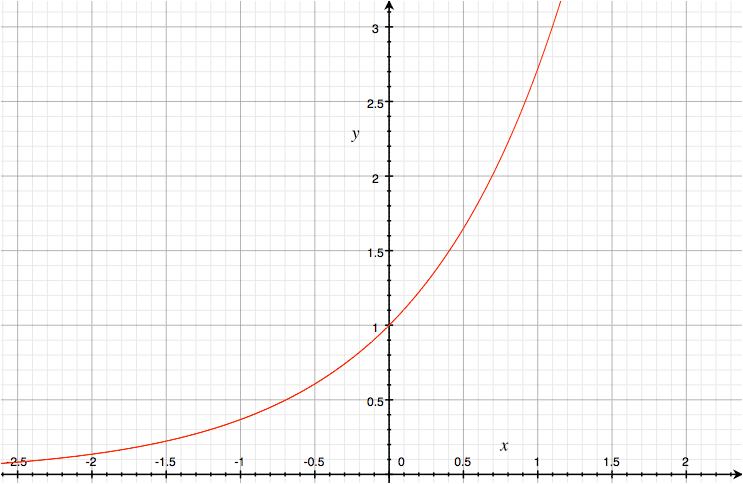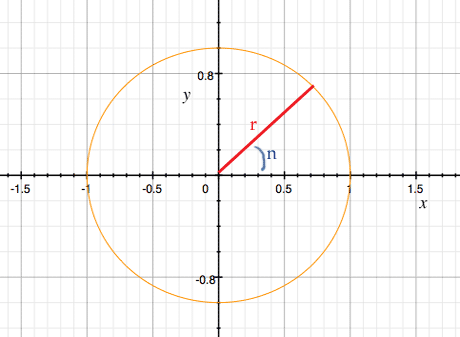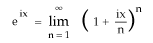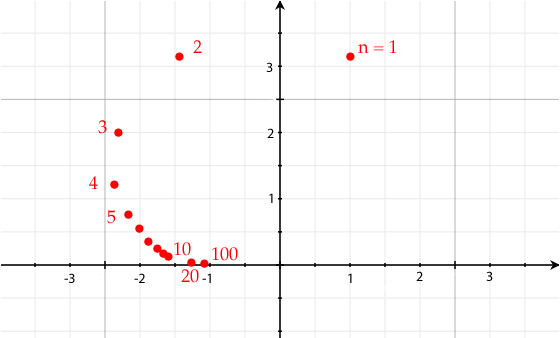I recently read Richard Feynman’s Lectures on Physics, vol. I, and he explained something that none of my professors ever did— an equation he calls an "amazing jewel".

So I thought I’d tell you about it. You can read Feynman yourself, of course— it’s chapter 22— but he was talking to college physics students, and I’m not going to assume you know anything more than high school math. Plus it’s no longer 1963, so I’ll assume you have a calculator, or access to Wolfram Alpha.

The derivative of ex

2.718281828459045235360287471352662497757247093699959574966967...
You’ll probably remember e as the base of natural logarithms. But before we get to logarithms, e has some remarkable characteristics.

To see one of them, let’s look at the function y = ex.What’s its derivative? We can guess by figuring out the slope at a few points, for instance at x = 1. The slope for a straight line between points x1, y1 and x2, y2 is (y2-y1)/(x2-x1).We can approximate the slope for the graph by picking two points very close together— say 1.0 and 1.0001. e1 is of course e ≅ 2.7182818, while e1.0001 is ≅ 2.7185537.

Note: We’re going to be seeing a lot of irrational numbers here. If you see ≅ (approximately equal), that’s a reminder that I’ve truncated the number, which really goes on forever.

So the slope is

(2.7185537 - 2.7182818) / (1.0001 - 1) ≅ 2.718417747
Which is close to e. Let’s try a better approximation of the slope, by choosing x2, y2 closer to 1:
(e1.000001 - e1)/ .000001 ≅ 2.718283188
Which is even closer to e. It’s beginning to look like the slope at e1 is e1, and in fact it is. You can verify all of these at Wolfram Alpha; e.g. copy and paste ((e^1.000001) - (e^1))/.000001. See what happens if you use closer and closer points.

Let’s try e0, whose value is 1.

(e0.000001 - e0)/.000001 ≅ 1.000000500
(e2.000001 - e2)/.000001 ≅ 7.389059793
(eπ+.000001-eπ)/.000001 ≅ 23.140704203
As these examples suggest, the slope of ex at any point is ex. That is, the derivative of ex is itself.
d/dx ex = ex
This is the only function of x whose derivative is itself. That makes ex very nice to work with in calculus— we’ll get back to that later.

Interested in defining e

e was originally calculated by Jacob Bernoulli by evaluating the limitYou have a dollar in an account that pays 100% interest in one year; after the year you’ll have \$2.

Suppose it’s 50% every 6 months, instead. Now you get \$1 × 1.5 × 1.5 = \$2.25.

If there are n intervals, the interest is 100%/n... that is, 1/n. Plus you keep the principal, so you have 1 + 1/n. E.g. for one month it’s 1 + 1/12 = 108.33% and after the year you have

(1 + 1/12)12 ≅ 2.61303529

Compounding daily, at 0.2739726%, gives us

(1+(1/365))365 ≅ 2.714567482
That’s starting to look like e, and if we keep making smaller and smaller divisions, that’s exactly where we end up.

Logarithms

To most people logarithms probably mean "mumble math something", so think of them as exponents, or order of magnitude. If you ask what order of magnitude a million is, you are in fact asking for log10 1,000,000 = 6.

We use logarithmic scales all the time; they’re great for reducing enormous ranges of huge numbers to easy-to-grasp small numbers. Some common examples:

• decibels: if a sound is ten times louder than another one, it’s 10 decibels higher
• the Richter scale: an earthquake 1.0 higher on this scale has 10 times the amplitude
• stellar magnitude: if one star is 100 times brighter than another, that’s 5 magnitudes
• the computing abbrevations kilo, mega, giga, tera, peta are a logarithmic scale— each step is 1024 times the last one
As a refresher, if ab = c, then a = b√c, and b = loga c. For instance, 103 = 1000, so 10 = 3√1000, and 3 = log10 1000.

Since we normally use base 10, base 10 logarithms are particularly easy.

• Log10 1000 = 3— just count the zeroes.
• Log10 1045 = 45.
• Log10 .001 = -3; count the places after the decimal point.
For centuries the most useful thing about logs was that they made for easy multiplication of large numbers. E.g. you want to multiply 3.14159265 by 2.7182818. That works out to
3.14159265
×2.7182818
-----------------
2513274120
3141592650
251327412000
629318530000
25132741200000
31415926500000
2199114855000000
6293185300000000
-----------------
8.539734123508770
Not difficult, but tedious and error-prone. But we can take advantage of the fact that
log ac = log a + log c
We can look up the logs in a table... tables to 14 decimal places have been available since 1620.
log10 3.14159265 ≅ .497149872
log10 2.7182818 ≅ .434294477
sum ≅ .93144434955
Now we can use the same table to find out what number this is the log of, which turns out to be ≅ 8.53973412.

Of course, these days we do nothing of the sort— we just use a calculator. But it was a huge time saver until the computer was invented.

(Some of you retro-geeks may say "No, no, people used slide rules." What do you think a slide rule is? Among other things, it’s a couple of logarithmic scales.)

One weird trick

There’s an interesting trick for taking powers of 1/n with higher and higher n. Let’s look what happens with e1/n, doubling n each time. (Or to put it another way, take e, then √e, then the square root of that, and so on.)
 1/n e1/n 1 + 1/n 1 2.7182818 2 1/2 1.648721271 1.5 1/4 1.2840254 1.25 1/8 1.133148453 1.125 1/16 1.06449446 1.0625 1/32 1.0317434075 1.03125 1/64 1.015747709 1.015625 1/128 1.007843097 1.0078125 1/256 1.003913889 1.00390625 1/512 1.00195503 1.001953125 1/1024 1.000977039 1.0009765625 1/2048 1.000488400 1.00048828125
If you’re thinking "Oooh, the limit of e1/n is 1", that’s correct, but that’s not the weird trick. The trick is that a good guess for e1/n is 1 + 1/n, and it’s an increasingly good guess as n gets huge.

We’ll use this trick in a clever way in a moment, but I’ll also point out two things.

1. This particular series is important if you want to calculate logarithms by hand. See Feynman’s lecture if you want to know how.
2. You may hear that e is a "convenient" base for logarithms. One thing that means is that this particular neat trick is made easier if your base is e. If it’s 10 instead, the trick involves another step: a good guess for 101/n is 1 + 2.3026 * (1/n). We get rid of the constant by using base-e logarithms.

Now, all of the algebraic operations— addition, multiplication, exponentiation, and their inverses subtraction, division, taking a root, finding a logarithm— should work on any number, so long as we’ve defined ‘number’ broadly enough. It turns out that complex numbers are as far as we need to go.

All of which means that a function like y = eix should be fine. Now, we can understand raising a number to a power n as multiplying it by itself n times. This is stretched by such ideas as raising it to a fraction or a negative number, and seems almost to break if the number is imaginary. But the algebra all works, so we’ll let the meaning take care of itself.

Still, how do we evaluate an expression like eix? Where do we even start?

We actually have a place to start— the observation from the last section that a good guess at ei/n is 1 + 1/n. Let’s assume that it’s true for complex numbers as well.

Let’s set n to 1024. That is, a good guess for ei/1024 is 1 + i/n = 1 + i/1024. That of course is the complex number 1 + .0009765625i.

That gives us one exponential of an imaginary number. (Or rather, an approximation; the actual value ≅ .999999523 + 0.00097656234i.

Given one value of eix, here are ways to get more; here’s one. Let’s try for i/512. We could use the 1 + 1/n trick, but it gets less accurate as 1/n gets bigger. Instead, let’s take

ei/1024 = 1 + .0009765625i
and square both sides. Now, (ab)c is always abc, so
(ei/1024)2 = e(i/1024)×2 = ei/512
For the other side, the general rule for complex numbers is
(a × bi)2 = (a × bi)(a × bi)
= a2 + 2abi + b2i2
= (a2 - b2) + 2abi
Here a = 1 and b = .0009765625, so we get
(1×1 - .0009765625×.0009765625) + 2×1×.0009765625×i
= 0.9999990463 + 0.001953125 i
Let’s see what ein looks like, as a function. (I’m just going to let Wolfram Alpha calculate it.) Here’s how it goes for several values of n.
 0 1 .2 0.9800666 + 0.1986693 i .4 0.921061 + 0.389418 i .6 0.825336 + 0.564642 i .8 0.696707 + 0.717356 i 1 0.540302 + 0.841471i 1.2 0.362358 + 0.932039 i 1.4 0.169967 + 0.985450 i 1.6 -0.0291995 + 0.999574 i 1.8 -0.227202 + 0.973848 i 2 -0.416147 + 0.909297 i 2.2 -0.588501 + 0.808496 i 2.4 -0.737394 + 0.675463 i 2.6 -0.856889 + 0.515501 i 2.8 -0.942222 + 0.334988 i 3 -0.989992 + 0.141120 i 3.2 -0.998295 - 0.0583741 i 3.4 -0.966798 - 0.255541 i
It looks like there’s some patterns here. All the values we’ve seen so far, both for the real part and the imaginary part, live between -1 and 1. Moreover, looking down the real column, we see the value smoothly going down; when it reaches -1, toward the end of our list, it inches back up again. The imaginary column goes up, reaches 1, and then declines.

Circling round the subject

Feynman points out something else of note. Simply from the definition of a complex number, we know that
ein = x + iy
Now, a square root always has two solutions, positive and negative. We usually say that √2 is 1.41421356, but we could equally well use -1.41421356. Likewise, if i is √(-1), so is -i. Replacing i with -i in an equation is called taking the complex conjugate, and it gives you another true equation:
e-in = x - iy
Now let’s multiply these equations together:
ein e-in = (x + iy)(x - iy)
Simplifying the left side:
ein e-in = ein - in = e0 = 1
And the right side:
(x + iy)(x - iy) = x2 +xyi -xyi - i2y2 = x2 + y2
So for any complex number x + iy that we found as a value of ein, we know that
x2 + y2 = 1
Does this ring any bells yet? That’s the formula for a circle, so circles are involved in this somehow.

The big reveal

Let’s just get a plot of ein. The red line is the real part, the blue line is the imaginary part.Those look like sine waves. And they are sine waves! In fact we can write
ein = cos n + i sin n
This is the equation Feynman calls the "amazing jewel" of mathematics. We’re just messing around with exponential functions, and we suddenly arrive at the trigonometric functions.

Rather than plotting ein, we can also plot x and y as n varies. We get this graph:That shouldn’t be surprising, since x2 + y2 = 1 is how you plot a circle. However, it gives us an alternate way of thinking about ein— we can think of n as an angle, as in the picture. The radius r is also shown, and of course it’s 1.

We can’t represent all complex numbers with ein... but we can represent them as rein. That is, an alternative representation of a complex number x + iy is rein.

Proofs

Maybe it looks like a sine wave but it really isn’t? Well, naturally, there are proofs of Euler’s formula. You can look here, or here, or here.

The problem is that to understand the proofs, you need far more math than is needed just to understand e and sin/cos! Euler himself used the limit expansions of en, of sine, and of cosine... but even though it’s true, it’s not intuitively obvious that cos x is the sum of -n/(2n)! × x2n.

Let’s take another approach, along the lines of "use Wolfram Alpha to evaluate limits for us until we’re satisfied we know where this is going". Above I mentioned that Bernoulli’s definition of e wasNow e = e1, so the general formula isx can be a complex number, so we can also writeLet’s try a particular value, namely x = π.

For n = 1, this is (1 + iπ/1)1 = 1 + i π.

You can enter (1 + (i*pi)/2)^2 into Wolfram Alpha, and keep going with higher and higher n’s. Wolfram Alpha will helpfully give you a plot of the results. Here’s how it goes:It looks like the limit is simply -1, and so it is. Wolfram Alpha will even do limits for you— cut and paste lim((1+(i*pi)/n)^n).

But does Euler’s formula

eix = cos x + i sin x
work for x = π? Cos π = -1 and sin π = 0, so yes. π is of course halfway round the unit circle.

Let’s try another number, say π/6, or 30 degrees. Give Wolfram Alpha lim((1+(i*pi/6)/n)^n) and you get ½√3 + ½ i. And this checks out: if you take sin(π/6) you get ½, and cos(π/6) = ½√3.

Now all you have to do is try out every other possible value of x from 0 to 2π. Any number you try, it’ll work.

An example

The good thing about learning your math from Feynman’s lectures is that you almost immediately get a practical application. The bad thing is that to understand the application requires not just following the physical analysis, but having a firm grounding in differential equations.

So to demonstrate the use of complex exponents of e, I’m just going to show one step in a longer derivation. Feynman doesn’t even bother to show the step— he says it’s evident "by inspection"— but I’ll take it a bit slower.

We have a harmonic oscillator (such as a weight held by a spring), driven by a force F, and resisted by friction. The equation is

d2x/dt2 + γ(dx/dt) + ω02x = F/m
F is itself defined as F0 cos(ωt + Δ). This makes for a pretty rough differential equation, but here’s where our trick comes in. We pretend that F isn’t a cosine but an exponential, and just ignore the imaginary part. So we use Feiωt for the force and xeiωt for the displacement. (The underlines are reminders that these are complex numbers.) Now the differential equation is
d2xeiωt /dt2 + γ(dxeiωt /dt) + ω02xeiωt = Feiωt/m
Recall that the derivative of et is itself. The derivative of eiωt is almost as easy: bring down the constants, giving iωeiωt. So the second term
γ(dxeiωt /dt)
is immediately converted to
γiωxeiωt
The first term is a double differentiation, which becomes a double multiplication by iω. That is,
d2xeiωt /dt2
becomes
(iω)2 xeiωt
which reduces to
2 xeiωt
So the differential equation vanishes, replaced by
2 xeiωt + γiωxeiωt + ω02xeiωt = Feiωt/m
If your math is rusty this may not have seemed like an improvement, but again, the clever bit is that we’ve replaced a differentiation with a multiplication, which is far less hairy.

Might as well take the next step: we can divide out eiωt from both sides:

2 x + γiωx + ω02x = F/m
Solving for x:
x = F/m(ω02 - ω2 + γiω)

This eiωt business isn’t just a quirk of weights on springs; it turns up all over. It’s a basic part of analyzing electric circuits; it comes up in tidal effects on the atmosphere; it pops up in nuclear physics. Nature is lazy, and uses the same damn equations for everything.